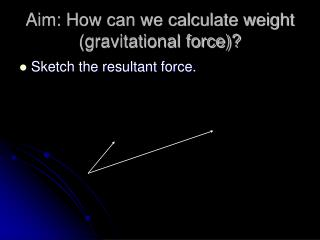DownloadDownload PresentationAim: How can we calculate weight (gravitational force)?

# Aim: How can we calculate weight (gravitational force)?

Télécharger la présentation## Aim: How can we calculate weight (gravitational force)?

- - - - - - - - - - - - - - - - - - - - - - - - - - - E N D - - - - - - - - - - - - - - - - - - - - - - - - - - -
##### Presentation Transcript

1. Aim: How can we calculate weight (gravitational force)? • Sketch the resultant force.

2. There are two types of forces. Contact and noncontact forces. • Contact forces: • Friction • Tension • Applied (push of pull) • Normal force • Air Resistance • Non Contact Forces: • Gravitational • Electrostatic • Magnetic

3. Mass-Force-Weight How can we define mass? Anything that has matter. (something that we can touch) Mass is constant no matter where the object is located in the universe. Mass is measured in kilograms. (kg) Mass is a scalar quantity. If my mass on Earth is 72 kg, then what is my mass on the moon? 72 kg

4. How can we define a force? • A force is a push or pull on a mass (object). • Force is a vector quantity. It needs both magnitude and direction. • Force is measured in Newtons. Abbreviation (N).

5. How can we describe weight? • Weight is a measurement of the gravitational force acting on an object. • Symbol for weight is Fg. Formula to calculate weight is • m stands for mass and g stands for acceleration due to gravity.

6. Example • Bob has a mass of 80 kg. Calculate his weight on the earth.

7. An 82 kilogram student weighs 1153.74 newtons. Determine the planet that the student is standing on.

8. How much does Bob weigh on the moon? • The acceleration due to gravity on the moon is 1/6th of Earth’s.

9. A person weighs1,826 Newtons on Jupiter determine the person’s weight? • Acceleration due to gravity on Jupiter is 23.12 m/s2.

10. Calculate the acceleration due to gravity.